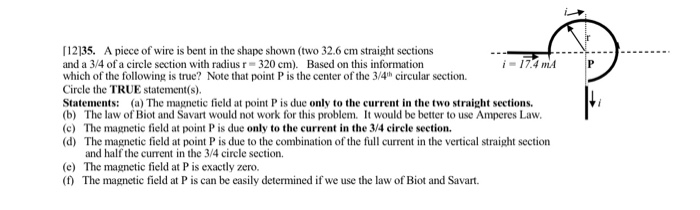# Р 35. A piece of wire is bent in the shape shown (two 32.6 cm straight...

###### Question:Р 35. A piece of wire is bent in the shape shown (two 32.6 cm straight sections and a 3/4 of a circle section with radius r - 320 cm). Based on this information 17.4 m which of the following is true? Note that point P is the center of the 3/4th circular section. Circle the TRUE statement(s). Statements: (a) The magnetic field at point P is due only to the current in the two straight sections. (b) The law of Biot and Savart would not work for this problem. It would be better to use Amperes Law. (c) The magnetic field at point P is due only to the current in the 3/4 circle section. (d) The magnetic field at point P is due to the combination of the full current in the vertical straight section and half the current in the 3/4 circle section (e) The magnetic field at P is exactly zero. (1) The magnetic field at P is can be easily determined if we use the law of Biot and Savart. Hi

#### Similar Solved Questions

##### Calculate the deflection of the rectangular wood beam at the load application point, 8 ft from...
Calculate the deflection of the rectangular wood beam at the load application point, 8 ft from A. Write you answer in inches to 2 decimal places given the wood modulus of elasticity, E is 1.1x103ksi. 600 lb A To in. B -X2 3 in. 8 ft - 4 ft -...